Previous:  Input files
Next: Routines
Contents

IV. MESH GENERATION

A. INTRODUCTION

As seen in the previous Section, the input to the EMAP4 code is a simple text file. This means that the EMAP4 code needs to generate its own mesh and store the mesh information. This problem is tackled in a very simple way. The user defines a coarse mesh in terms of cubes (hexahedra). EMAP4 takes this coarse mesh made up of hexahedra and divides each cube into five tetrahedra. These tetrahedra form the basic elements in the formulation. An important advantage of FEM is that the tetrahedral elements need not all be the same size and shape. This is exploited by using a non-uniform mesh as discussed in Section IV.C. The next section explains how the information about the mesh is stored, how the hexahedra are divided into tetrahedra and how the nodes and edges are numbered.

B. STORAGE OF MESH INFORMATION

The mesh information is stored using a number of variables in EMAP4. The system of storage used here makes it easy for the main program to retrieve information about the mesh. Every tetrahedron has four nodes and six edges. Figure 2 and Table II explain how the nodes and edges in a tetrahedron are numbered. Each edge has a specific direction associated with it as shown in the figure. Each hexahedron is divided into five tetrahedra as shown in Figure 3. This can be done in two different ways . To make sure that the diagonal edges line up the same way when the hexahedra are pieced together, adjacent hexahedra are divided into tetrahedra in different ways (from the figure it can be seen that the diagonal edges on opposite sides are oriented differently). Each hexahedron has eight nodes and eighteen edges. While dividing a hexahedron into five tetrahedra, each tetrahedron takes six edges and eight nodes from the eighteen and eight respectively in the hexahedron.

One of the aspects in the mesh generator is the difference between local and global numbering. The global node numbering (ordering) is as follows. It starts off from x=y=z=0, first finishes up the z=0 plane and then goes on to higher values of z. In the z=0 plane, it starts off at the y=0 line and goes on to higher y. The edge numbering follows in the same general direction. The edges are numbered first starting from the z=0 plane and then the edges in between z-planes are numbered and so on. The local numbering is shown in Figure 4. Global numbering for a simple configuration is given in Figure 5.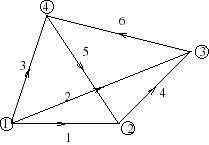Figure 2: Tetrahedron edge definition.

Table II. Edge definition in a tetrahedron.

 Edge number Start node End node 1 1 2 2 1 3 3 1 4 4 2 3 5 4 2 6 3 4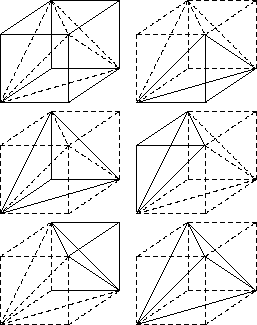Figure 3: Division of a hexahedron into five tetrahedra.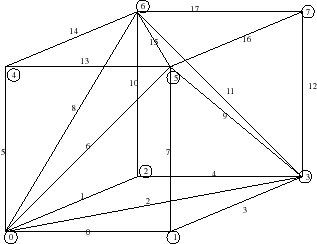Figure 4: Local numbering of hexahedron edges.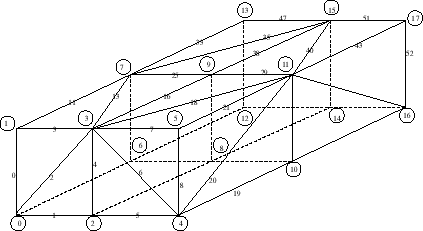Figure 5: Global edge and node numbering systems.

The mesh information is stored using the following variables:

TotNodeNum : Total number of nodes.

TotEdgeNum : Total number of edges.

HexNum : Total number of hexahedra.

HexNode [HexNum:8]: Stores the global node number for each of the local nodes. HexEdgeNum [HexNum:18]: Stores the global edge number for each of the local edges.

NodeCord[TotNodeNum:3]: This stores the global coordinates of all the global nodes.

TetGlobalEdgeEnds : Stores the end nodes of the six edges in each tetrahedron. This variable distinguishes between the two ways of hexahedron division.

TetGlobalNodeNum : This is similar to HexNode for each tetrahedron.

TetEdgeNum : This is similar to HexEdgeNum for each tetrahedron.

C. NON-UNIFORM MESH

An important advantage of FEM, as compared to FDTD is that the mesh doesn't need to be uniform. This is useful when modeling structures like traces or wires etc. Non-uniform meshes are used in situations where there are large field changes in some places and small field changes at other places. EMAP4 uses a default mesh made up hexahedra. The celldim keyword allows the user to vary the size of the hexahedra. The dielectric slab in Figure 1 has a length of 21 cm in the x-direction, 24 cm in the y-direction and 32 cm in the z-direction. The following example demonstrates how a non-uniform grid is specified using the keyword "celldim",

celldim 0 4 x 1 cm

celldim 4 8 x 2 cm

celldim 0 1 y 3 cm

celldim 1 2 y 2 cm

celldim 2 3 y 1 cm

celldim 0 3 z 2 cm

celldim 3 5 z 1 cm

As shown in Figure 6, the length along the x-axis of each brick (hexahedron) is 1 cm in the interval [1, 4], and 2 cm in the interval [4, 12]. The length along the y-axis of each brick is 3 cm in the interval [0, 3], and 2 cm in the interval [3, 5], and 1 cm in the interval [5, 6]. The segment length along the z axis of each brick is 2 cm in the interval [0, 6], and 1 cm in the internal [6, 8]. The slab is divided into 8´ 3´ 5=120 hexahedra. The coordinates are in terms of the number of bricks (hexahedra).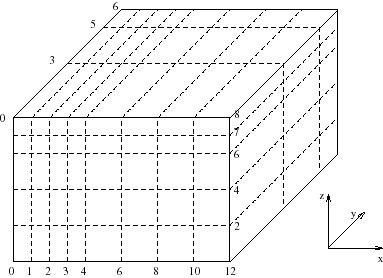Figure 6: A nonuniform mesh.